# Erdös-Ginzburg-Ziv theorem

Jump to: navigation, search

EGZ theorem

If $m$ is a positive integer and $a_1,\ldots,a_{2m-1}$ is a sequence of elements from the cyclic group $\mathbb{Z}_m$, then there exists a set $I\subseteq \{1,\ldots,2m-1\}$ of cardinality $m$ such that $\sum_{i\in I}a_i=0$. This theorem was first shown in [a5].

## Related theorems.

Looking at a sequence ofzeros andones one sees thatcannot be replaced by a smaller number. This motivates the definition of the Erdös–Ginzburg–Ziv constant for an arbitrary Abelian group, as follows. Ifis an Abelian group, thenis the minimum integersuch that every sequence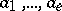of elements fromcontains a subsequence of cardinality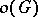, the order of, that adds up to. It can be proven that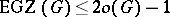and equality holds if and only if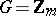. This result and the observation above led to the following two directions of investigation:

i) To find bounds, or possibly determine,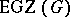for groups other than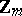in terms ofand other group invariants. Recent results in this direction were obtained by Y. Caro and Weidong Gao.

ii) To find or estimate the minimum integer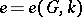such that every sequenceof elements fromcontains a subsequence of cardinalitythat adds up to, provided one knows that there are at leastdistinct elements in the sequence. Recent results in this direction are due to A. Bialostocki, P. Dierker, Y.O. Hamidoune, and M. Lotspeich. A breakthrough in this direction was achieved after the recent proof of the long standing Erdös–Heilbronn conjecture (cf. also Erdös–Heilbronn problem). Along a different line, J.E. Olson extended the definition of theconstant to non-Abelian groups and proved thatstill holds.

## Outline of developments.

There are several reasons why this theorem has recently (1996) drawn much attention.

Quite unexpectedly, N. Alon and M. Dubiner [a1] have shown that the theorem follows from several deeper results in algebra and number theory, establishing interesting links.

The theorem has many possible generalizations, some of which have been proved and others are easy to state as fundamental open problems, see [a4] and the conjectures below.

The theorem motivated the development of what is called a zero-sum Ramsey theory: If the sequence in the theorem consists only of the elementsand, then its proof follows from the pigeon hole principle (cf. Dirichlet principle), hence the theorem can be viewed as a generalization of this principle. Consequently, since Ramsey theory (cf. also Ramsey theorem) is a development of the pigeon hole principle, there is a clear motivation to develop from the EGZ theorem a zero-sum Ramsey theory along the lines of traditional Ramsey theory. While in Ramsey theory one looks for monochromatic configurations, in zero-sum Ramsey theory the colours are elements of a group and one looks for zero-sum configurations. Zero-sum Ramsey theory generalizes many results of the traditional Ramsey theory and leaves many open problems.

## Proofs.

In [a1] five proofs for the EGZ theorem have been given. In all these proofs it is assumed thatis a prime number, since the transition to a non-prime is a simple induction. The original proof is based on the Cauchy–Davenport theorem from elementary additive number theory; two other proofs use the Fermat little theorem, along with a counting argument and a lemma concerning permanents, respectively. A fourth proof uses the Chevalley–Warning theorem about zeros of polynomials over a finite field. Most interesting is the proof that uses knowledge of the Davenport constant of an Abelian-group, determined by Olson. Letbe a finite Abelian group. The Davenport constant of, denoted by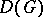, is the minimalsuch that every sequence ofelements fromcontains a subsequence that adds up to.

## Generalizations and analogues.

Clearly, ifis cyclic of order, then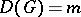. An interesting relation betweenand the EGZ theorem is Weidong's generalization of the EGZ theorem: Letbe a sequence of elements from an Abelian group. If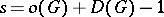, then there exists a setof cardinalitysuch that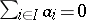.

The following two conjectures are other possible generalizations of the EGZ theorem.

### Conjecture 1.

Letandbe positive integers. If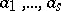is a sequence of elements from the cyclic group, then it contains at least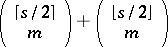subsequences ofelements that add up to.

If one takesin this conjecture, then the EGZ theorem follows.

### Conjecture 2.

Letbe a positive integer and letbe a sequence of elements from the cyclic groupwhose sum is. If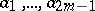is a sequence of elements from, then it contains a subsequence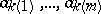such that the sequencecan be reordered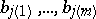such that.

If one takes,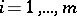, in this conjecture, then the EGZ theorem follows.

Conjecture 1 was proven by M. Kisin for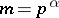and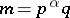, whereandare distinct prime numbers and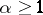. In [a7], Z. Füredi and D.J. Kleitman confirmed Conjecture 1 asymptotically for every positive integer. Conjecture 2 can be easily proven forprime. Both conjectures illustrate the general difficulty that exists in this area for handling the non-prime case.

There are many related problems to the EGZ theorem. The following conjecture was raised by H. Harborth and some progress was made by Alon and Dubiner. It illustrates that certain problems are open, even for primes.

### Conjecture 3.

Ifis a prime and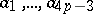is a sequence of elements from the group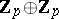, then there exists a subsequence ofelements whose elements add up to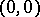.

A sequence containing only the elements,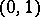,, and, where each element appears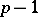times, implies that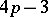can not be replaced by a smaller number.

## Zero-sum Ramsey theory.

This theory was first introduced in [a3]. Today it includes many results on zero-sum Ramsey numbers for graphs. Recent developments by Bialostocki, P. Erdös, H. Lefmann, and D. Schaal deal with zero-sum solutions to systems of equations and inequalities over the integers. Surveys of this can be found in [a2] and [a4]. The following theorem from zero-sum Ramsey theory, proved in [a6] and [a8], generalizes in the sense of the EGZ theorem the folkloristic fact that in every-colouring of the edges of a complete graph there is always a monochromatic spanning tree: If each edgeof the complete graph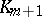(cf. also Graph theory) is assigned an element from the cyclic group, say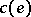, then there exists a spanning treeofwith edges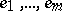such that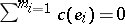.